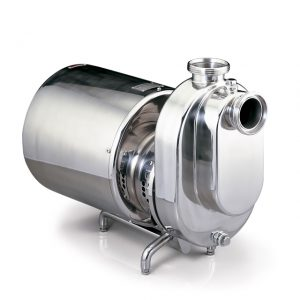# NPSH of Sanitary PumpsPosted by

A specific parameter of the pumps is the NPSH, namely Net Positive Suction Head. Consider that the suction of a pump takes place due to pressure difference, therefore under normal operating conditions, if a pump has to suck from a free surface lower than the pump itself, it will create a depression in the mouth of suction such as to raise the fluid of a height equal to the difference in height.Under normal conditions, the standard atmospheric pressure is equal to the pressure of 10.332 meters of water, so at most, ideally creating vacuum with the sanitary pump at the suction mouth, the water can rise, from the free surface pushed by the atmospheric pressure along the suction pipe, up to a height of 10.332 meters. In practice, considering the pressure drops in the ducts, the maximum rising height of the fluid will be lower.

So the maximum difference in height, from the free surface, from which it is possible to theoretically pump water is equal to 10.332 meters. In the case where the liquid to be pumped is mercury (which has a density of 13579 kg/m3), the theoretical maximum difference in height would be 0.76 meters. In reality the fluid will have a vapor pressure higher than 0, so, by pumping water and raising it from 10.332 meters, this would boil in the suction pipe.